## Monday, June 17, 2013

### I Need To Find The Event Horizon

...for my office.

Shouldn't be too hard to find the Swarzchild Radius...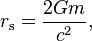$r_\mathrm{s} = \frac{2Gm}{c^2},$
where: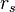$r_s\!$ is the Schwarzschild radius;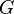$G\!$ is the gravitational constant;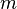$m\!$ is the mass of the object;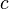$c\!$ is the speed of light in vacuum.
The proportionality constant, 2G/c2, is approximately 1.48×10−27 m/kg, or 2.95 km/solar mass.
An object of any density can be large enough to fall within its own Schwarzschild radius,$V_s \propto \rho^{-3/2},$
where: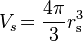$V_s\! = \frac{4 \pi}{3} r_\mathrm{s}^3$ is the volume of the object;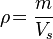$\rho\! = \frac{ m }{ V_s\! }$ is its density.

Because weird shit keeps accumulating inside my office.

To wit:
The NASA Surveyor 2 probe, supposedly crashed south of the Copernicus crater on the moon September 20 of 1966, has mysteriously appeared in my office.WTF, man?

TBG- ΜΟΛΩΝ ΛΑΒE